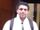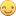## IF THEN statement with a combination of variables

Hi!

I'm working with a data set and I'm trying to see if any of the pregnancy responses are invalid based on gender (i.e. male participants should not be recorded as having ever been pregnant).

The values are as follows:

GENDER

M=Male

F= Female

PREGNANCY

0=never been pregnant

1=has been or is pregnant

9=not available

How do I write an IF THEN statement where if the participant is a man and has been pregnant than his category is 0 but any other combination of the two variables is category 1?

1 ACCEPTED SOLUTION

Accepted Solutionsnovinosrin
Tourmaline | Level 20

## Re: IF THEN statement with a combination of variables

constants are case sensitive while variable operands are case insensitive

IF pregnancy=1 AND gender=M THEN cat=0;

should be

IF pregnancy=1 AND gender='M' THEN cat=0;

change accordingly everywhere

6 REPLIES 6

## Re: IF THEN statement with a combination of variables

@MBlack732 wrote:

Hi!

I'm working with a data set and I'm trying to see if any of the pregnancy responses are invalid based on gender (i.e. male participants should not be recorded as having ever been pregnant).

The values are as follows:

GENDER

M=Male

F= Female

PREGNANCY

0=never been pregnant

1=has been or is pregnant

9=not available

How do I write an IF THEN statement where if the participant is a man and has been pregnant than his category is 0 but any other combination of the two variables is category 1?

Just put your last sentence in code. You have already formulated the statement, only using non-SAS syntax.

## Re: IF THEN statement with a combination of variables

I tried this code:

IF pregnancy=1 AND gender=M THEN cat=0;
ELSE IF pregnancy=9 and gender=M THEN cat=0;
ELSE IF pregnancy=9 and gender=F THEN cat=0;
ELSE IF pregnancy=1 and gender=F THEN cat=1;
ELSE IF pregnacy=0 and gender=F THEN cat=1;
ELSE IF pregnancy=0 and gender=M THEN cat=1;

and my log resulted in this error message:

206 IF pregnancy=1 AND gender=M THEN cat=0;
--
180

ERROR 180-322: Statement is not valid or it is used out of proper order.

207 ELSE IF pregnancy=9 and gender=M THEN cat=0;
----
180

ERROR 180-322: Statement is not valid or it is used out of proper order.

208 ELSE IF pregnancy=9 and gender=F THEN cat=0;
----
180

ERROR 180-322: Statement is not valid or it is used out of proper order.

209 ELSE IF pregnancy=1 and gender=F THEN cat=1;
----
180

ERROR 180-322: Statement is not valid or it is used out of proper order.

210 ELSE IF pregnacy=0 and gender=F THEN cat=1;
----
180

ERROR 180-322: Statement is not valid or it is used out of proper order.

211 ELSE IF pregnancy=0 and gender=M THEN cat=1;
----
180

ERROR 180-322: Statement is not valid or it is used out of proper order.novinosrin
Tourmaline | Level 20

## Re: IF THEN statement with a combination of variables

constants are case sensitive while variable operands are case insensitive

IF pregnancy=1 AND gender=M THEN cat=0;

should be

IF pregnancy=1 AND gender='M' THEN cat=0;

change accordingly everywhere

## Re: IF THEN statement with a combination of variables

Was that code within a data step? I think that's your issue.

data want; *output data set name;
set have; *input data;

IF statements (corrected for character variables)

run;

## Re: IF THEN statement with a combination of variables

Using @MBlack732 If statements with quotes and correct spelling for varables with samename this works but you want to rethink your formats for the men.  Also note I used the upcase on gender incase a user could enter upper or lower case values.

updated for @novinosrin to be shorter

``````/* Notes
GENDER
M=Male
F= Female

PREGNANCY
0=never been pregnant
1=has been or is pregnant
9=not available
*/

data have;
input name \$ pregnancy \$ gender \$;
cards;
Mary 1 F
Mike 1 m
John 0 M
Ed 0 M
Ann 9 F
Gwen 0 F
;
data want;
set have;
gender = upcase(gender);
if gender = 'M' and pregnancy = '1' then cat=0;        else cat = 1;
run;``````novinosrin
Tourmaline | Level 20

## Re: IF THEN statement with a combination of variables

Hi @VDD Pleasing to note you took time to write down the IF THEN statements.

Just a nit and hint, try to see if those IF THEN's can be further shortenedDiscussion stats
• 6 replies
• 1384 views
• 0 likes
• 5 in conversation